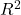# Estimating proportion of explained variation for an underlying linear model using logistic regression analysis

Eighttype statistics proposed to use in logistics regression analysis are evaluated
based upon their ability to predict the proportion of explained variation
() for an underlying linear model with latent scale continuous dependent variable.
Functional relationships between these statistics are also studied. Predictive
quality of these statistics depends mainly upon the proportion of success in the
sample and, the quantity to be predicted. We found(Hagle and Mitchell (1992)) to be numerically closest to the underlying . There is a one-to-one
correspondence between the likelihood basedstatistics, some of which have
been considered independent until recently.

Fulltext